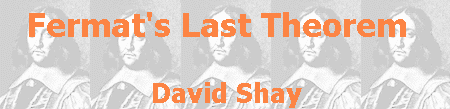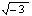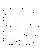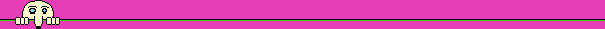## Proofs for special cases

One of the methods used also by mathematicians in their struggle with hard problems is "Divide and Conquer" - splitting the problem into sub-problems that are easier to handle. This tactic appears in connection with FLT already in Fermat's writings, which contain a kernel of a proof for the special case n=4. This is the only proof in number theory that Fermat left (it had space in the margin of Diophantus' book), and it uses the infinite descent method. Fermat also dealt with the special case n=3, as we learn from his letter to Christian Hugeness, but, habitually, he did not leave a proof for this case.

The proof for the special case n=3 was found one hundred years later by Leonhard Euler, the greatest mathematician of the eighteenth century. In number theory, Euler is the follower of Fermat: many theorems stated by Fermat were proven by Euler. In 1753 Euler wrote to Christian Goldbach about the proof, but he did not publish it. In 1770, in his book Anleitung zur Algebra (A Guide to Algebra), Euler gave a proof for this case. This proof was slightly different from the one he gave to Goldbach, and in it Euler used algebraic numbers, which are created by adjoining numbers of the form, for example, to the group of whole numbers, creating numbers of the form a+b, where a and b are integers. Although these numbers are not integers, Euler found them useful to solve Diophantine equations.

Euler proofs use the infinite descent method, strengthening Fermat's claim that this method "will lead to marvelous advancement in the theory of numbers". Except the proof technique, the proof for the case n=3 is different from the one for the case n=4, and this explains why, in his letter to Goldbach, Euler said that he did not see any hope to generalize those two special proofs into a general proof. Desperately, Euler asked to make a thorough search in Fermat's home, in hope to find a lead to the missing proof.

The mail connection between Euler and Goldbach lasted 35 years, and is an important source of information about Euler's work. In the beginning of this correspondence, in 1729, Goldbach motivated Euler to deal with Fermat's work. In a letter from 1748, Euler mentioned for the first time Fermat's Last Theorem ("a very nice theorem", he wrote later). Goldbach's Conjecture appeared in a letter from 1742. This conjecture is the main direct contribution of Goldbach to the development of mathematics. His indirect influence, as a friend to Euler, is much more important. In 1843, sixty years after Euler death, his grand grandson published the full correspondence.The proof for the case n=5, in a way that was an enlargement of the way Euler used for his proof of the case n=3, was created by two partners: the German Peter Gustav Lejeune Dirichlet, the pupil and friend of Gauss, who was 20-year-old, and the French Adrien Marie Legendre, one of the leaders of the French Academy of Sciences and follower of Fermat and Euler in building the foundations of number theory, who was 73-year-old. Dirichlet divided the proof needed for this case into two cases, and in July 1825 he presented to the Academy of Sciences a proof to one of those cases. Two months later, Legendre published, as an appendix to his successful book Théorie des nombres (Number Theory), a proof for the second case, completing Dirichlet's proof. Two months later, Dirichlet supplied a nicer proof for the second case, a kind of natural continuation of his proof for the first case.

Fourteen years later, Gabriel Lamé proved the theorem for the case n=7. Why did Lamé skip the proof for n=6? If the numbers A, B and C are a solution to Fermat's equation for the power of 6, namely A6+B6=C6, then the numbers A2, B2 and C2 are a solution to the power of 3, contradictory to the proof that FLT is true for this power. Generally, if we had proven FLT to any power k, than the theorem is valid to all the multiples of k. The reason for this is that if the numbers A, B and C are a solution for the power mk, then the numbers Am, Bm and Cm are a solution to the power of k, contradictory to the proof that FLT is true for this power.

 This proof technique is called proof by contradiction (or Reductio ad absurdum, if you prefer to say it in Latin). Fermat's infinite descent method is a special type of this technique.

 It follows from this proof that it is enough to prove FLT just for powers that are primes greater than 2, since each other power is a multiple of these primes (except 4, but for this power the theorem has already been proven by Fermat). It is a big step toward reduction of the scope of the problem, but it is still leaving us with the need to prove FLT for an infinite set of powers. An elegant proof of the infinitude of primes appears in Euclid's book, the Elements. In the first two centuries that passed since Fermat wrote his theorem, number theory had developed enormously. After the pioneer work of Fermat, Euler and Legendre, in 1801 Carl Friedrich Gauss published his book, Disquisitiones arithmeticae (Arithmetic Research), which turned number theory from a collection of isolated results into a systematic mathematical theory. Gauss, one of the greatest mathematicians of all times, called number theory "The Queen of Mathematics". Gauss was very pedantic about the quality of works he gave for publication, and many of his achievements were published many years after they were accomplished. A proof by Gauss for the case n=3 of FLT, different from that of Euler but also using the infinite descent method, was published after his death.